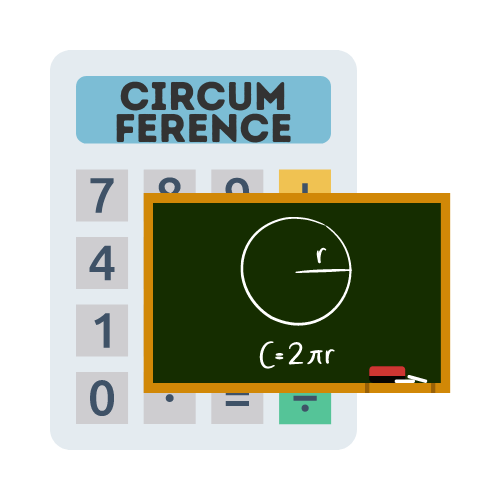# Circumference Calculator

## Free circumference calculator to measure the perimeter of a circle

The circumference is:

## What is the circumference of a circle?

The circles circumference is the distance around the circle. You can think of it as a perimeter of the circle, the distance of the outer line that goes the whole way around the circle.

## How to find the circumference of a circle?

In order to calculate the circumference of a circle, you will need to already know the radius of the circle. Once you have the radius value, you can make a simple calculation of 2 x π (3.14) x radius to get the circumference value.

## Why do we calculate the circumference?

It is useful to calculate the circumference as it enables us to determine other values of the circles measurements. For example, if we know the circumference, then we can use that to calculate the radius of the circle or determine the area of circular area or cylinder.

The circumference is used regularly when calculating area and shape. It is also used regularly in everyday life and has many applicable use cases.

## When to use circumference?

The circumference is commonly used in Mathematics, such as measuring shapes and calculating various forms of geometry. However it is also used in the real world with physical objects.

Measuring the circumference makes it possible to calculate physical properties of objects, such as the area of the space contained within a circular object or the volume it consumes.

## What circumference means

The length of a circle's edge in straight lines is its circumference. The term "perimeter" is only used to refer to polygons, but it means the same thing as the perimeter of a geometric figure.

Frequently, circumference is spelt circumfrence.

## Who first created circumference

Greek mathematician Eratosthenes was the first to determine the circumference of the Earth in 240 B.C. He found that at the summer solstice, objects in a city on the Northern Tropic do not cast a shadow at midday, but they do in a more northern position. He was able to determine the circumference of the Earth using this information and the distance between the spots.

## What is the formula for circumference?

The formula for the circumference of a circle is `C=2πr`:

`Circumference = 2 x pi x radius`

The formula for circumference can be utilized by multiplying 2 x pi (3.14) and then multiplying the result by the radius.

## What is the measurement for a circle's circumference?

The circumference of a circle, which is equal to the length of its edge in linear distance, denotes a length. Therefore, for the metric system, the most popular units for measuring a circle's circumference are millimeter, centimeter, and meter, while for the imperial system, they are inch, foot, and yard.